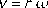A point on a spinning object moves in a circle. The speed of the point is the radius of that circle times the magnitude of the angular velocity (where the magnitude of the angular velocity is how fast the object is spinning).

Note: The point "goes" and the object "spins". This equation emphasizes the fact that omega does not have units of m/s. If omega had units of m/s, with r in meters, you would get v in units of meters-squared-per-second. But those are not the units for speed. Omega has units of radians-per-second where the radians can be thrown out yielding v in the correct units, m/s.

Pitfall Avoidance Note: Omega must be in units of radians-per-unit-of-time (usually radians-per-second) for this equation to hold true.